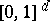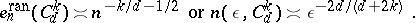# Curse of dimension

curse of dimensionality

Many high-dimensional problems are difficult to solve for any numerical method (algorithm). Their complexity, i.e., the cost of an optimal algorithm, increases exponentially with the dimension. This is proved by providing exponentially large lower bounds that hold for all algorithms. Many problems suffer from the curse of dimension. Examples include numerical integration, optimal recovery (approximation) of functions, global optimization, and solution of integral equations and partial differential equations. It does not, however, hold for convex optimization and ordinary differential equations. The term "curse of dimensionality" seems to go back to R. Bellman [a2].

The investigation of the curse of dimension is one of the main fields of information-based complexity, see also Optimization of computational algorithms. Whether a problem suffers from the curse of dimension depends on the exact definition of the norms and function classes that are studied. Some high-dimensional problems can be computed efficiently using the Smolyak algorithm.

The curse of dimension typically happens in the worst-case setting, where the error and cost of algorithms are defined by their worst performance. One can hope to break the curse of dimension by weakening the worst-case assurance and switching to the randomized setting (see also Monte-Carlo method) or to the average-case setting (see Bayesian numerical analysis).

Below, results for numerical integration are discussed in more detail. Many of the results are similar for other problems such as approximation (optimal recovery) of functions, solution of partial differential equations and integral equations.

## Worst-case setting.

Consider a quadrature formula of the form

\begin{equation} \tag{a1} Q _ { n } ( f ) = \sum _ { i = 1 } ^ { n } c _ { i } f ( x _ { i } ) \end{equation}

with $c _ { i } \in \bf R$ and $x _ { i } \in [ 0,1 ] ^ { d }$ for the approximation of the integral

\begin{equation*} I _ { d } ( f ) = \int _ { [ 0,1 ] ^ { d } } f ( x ) d x. \end{equation*}

Let $F _ { d }$ be a class of integrable functions on. Below, $F _ { d }$ will be the unit ball in a suitable Banach space. The (worst case) error of $Q _ { n }$ on $F _ { d }$ is defined by

\begin{equation*} e ( Q _ { n } , F _ { d } ) = \operatorname { sup } \{ | I _ { d } ( f ) - Q _ { n } ( f ) | : f \in F _ { d } \} \end{equation*}

and one also defines

\begin{equation*} e _ { n } ( F _ { d } ) = \operatorname { inf } _ { Q _ { n } } e ( Q _ { n } , F _ { d } ). \end{equation*}

The number $e _ { n } ( F _ { d } )$ is the (worst case) error of the optimal cubature formula (for the class $F _ { d }$) when at most $n$ sample points are used. It is known that formulas of the form (a1) are general enough. Indeed, non-linear methods and adaptive quadrature do not yield better estimates if $F _ { d }$ is convex and symmetric. Hence, one may say that the-complexity of the problem is given by the number

\begin{equation*} n ( \epsilon , F _ { d } ) = \operatorname { min } \{ n : e _ { n} ( F _ { d } ) \leq \epsilon \}. \end{equation*}

Much is known concerning the order of $e _ { n } ( F _ { d } )$ and of related numbers (such as widths and-entropy (see also Width;-entropy; or [a9]), for many different $F _ { d }$). Classical results on the order, see (a2) and (a3) below, contain unknown constants that depend on $d$ and therefore cannot always answer the question whether there is a curse of dimension or not.

Two specific results on the order of $e _ { n } ( F _ { d } )$ are as follows. Let $C ^ { k } ( [ 0,1 ] ^ { d } )$ be the classical space with norm

\begin{equation*} \| f \| = \sum _ { | \alpha | \leq k } \| D ^ { \alpha } f \| _ { \infty }, \end{equation*}

where $\alpha \in \mathbf{N} _ { 0 } ^ { d }$ and $| \alpha | = \sum _ { l = 1 } ^ { d } \alpha _ { l }$, and let $C _ { d } ^ { k }$ be the unit ball of $C ^ { k } ( [ 0,1 ] ^ { d } )$. It is known [a1] that

\begin{equation} \tag{a2} e _ { n } ( C _ { d } ^ { k } ) \asymp n ^ { - k / d } \text { or } n ( \epsilon , C _ { d } ^ { k } ) \asymp \epsilon ^ { - d / k }. \end{equation}

Hence, integration for the class $C _ { d } ^ { k }$ suffers from the curse of dimension. Define now a tensor product norm by

\begin{equation*} \| f \| ^ { 2 } = \sum _ { \alpha _ { l } \leq k } \| D ^ { \alpha } f \| ^ { 2 _{L _ { 2 }}}, \end{equation*}

for $f : [ 0,1 ] ^ { d } \rightarrow \bf R$, where the sum is over all $\alpha \in \mathbf{N} _ { 0 } ^ { d }$ with $\alpha _ { l } \leq k$ for all $l$. This is the space of functions with bounded mixed derivatives. Let $H _ { d } ^ { k }$ be the respective unit ball. It is known [a8] that

\begin{equation} \tag{a3} e _ { n } ( H _ { d } ^ { k } ) \asymp n ^ { - k } \cdot ( \operatorname { log } n ) ^ { ( d - 1 ) / 2 }. \end{equation}

This result of course implies an upper bound

\begin{equation} \tag{a4} e _ { n } ( H _ { d } ^ { k } ) \leq c _ { k , d , \delta} \cdot n ^ { - k + \delta } , \forall n, \end{equation}

for all $\delta > 0$, hence the order of convergence does not depend on $d$. Nevertheless, this problem suffers from the curse of dimension, see [a13].

The lack of the curse of dimension is related to the notion of tractability for continuous problems which was studied by H. Woźniakowski and others, see [a6], [a7], [a11], [a12]. The problem is tractable (in $d$ and) if

\begin{equation*} n ( \epsilon , F _ { d } ) \leq K . d ^ { p } . \epsilon ^ { - q } , \quad \forall d = 1,2 , \dots , \forall \epsilon \in ( 0,1 ], \end{equation*}

for some positive constants $K$, $p$ and $q$.

There are general results characterizing which problems are tractable. In particular, this holds for weighted tensor product norms, see [a7], [a13], under natural conditions on weights. The weighted norms correspond to the common fact that for high-dimensional problems usually some variables are "more important" than others. The classical discrepancy also corresponds to a space of functions with bounded mixed derivatives, see [a4]. The respective integration problem is tractable, with upper bounds of the form $n ( \epsilon , F _ { d } ) \leq \kappa \cdot d \cdot \epsilon ^ { - 2 }$, see [a13].

## Randomized setting.

In the randomized setting one considers quadrature formulas (a1) where the sample points $x_{i}$ and the coefficients $c_{i}$ are random variables. The error of a randomized method can be defined by

\begin{equation*} e ^ { \operatorname { ran } } ( Q _ { n } , F _ { d } ) = \operatorname { sup } \{ \mathsf{E} ( | I _ { d } ( f ) - Q _ { n } ( f ) | ) : f \in F _ { d } \}, \end{equation*}

where $\mathsf{E}$ stands for mathematical expectation, and one also considers the minimal randomized error $e _ { n } ^ { \operatorname {ran} } ( F _ { d } ) = \operatorname { inf } _ { Q _ { n } } e ^ { \operatorname {ran} } ( Q _ { n } , F _ { d } )$, see [a1], [a3], [a5], [a10], [a12], where more general notions are discussed. Instead of (a2), one now hasThe classical Monte-Carlo method gives an upper bound $n ^ { - 1 / 2 }$ for all these spaces, without an additional constant. Hence the problem is tractable in the randomized setting. Many other problems which are intractable in the worst-case setting stay intractable in the randomized or average-case setting.

For results concerning the average-case setting see Bayesian numerical analysis.

How to Cite This Entry:
Curse of dimension. Encyclopedia of Mathematics. URL: http://encyclopediaofmath.org/index.php?title=Curse_of_dimension&oldid=50805
This article was adapted from an original article by Erich Novak (originator), which appeared in Encyclopedia of Mathematics - ISBN 1402006098. See original article